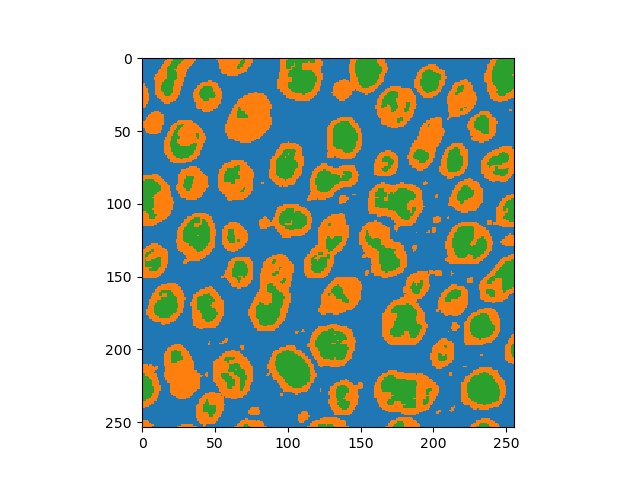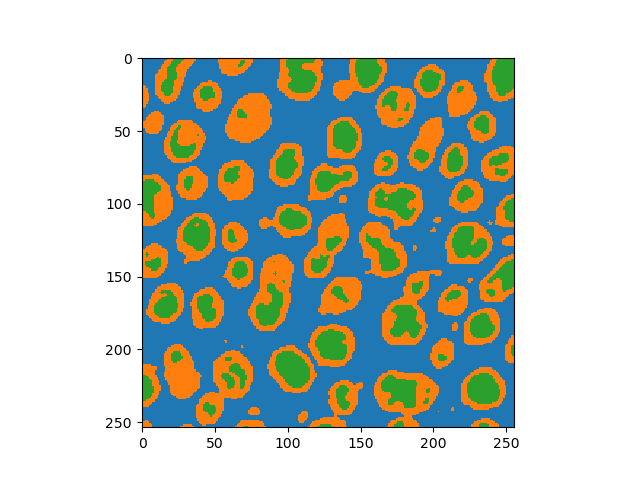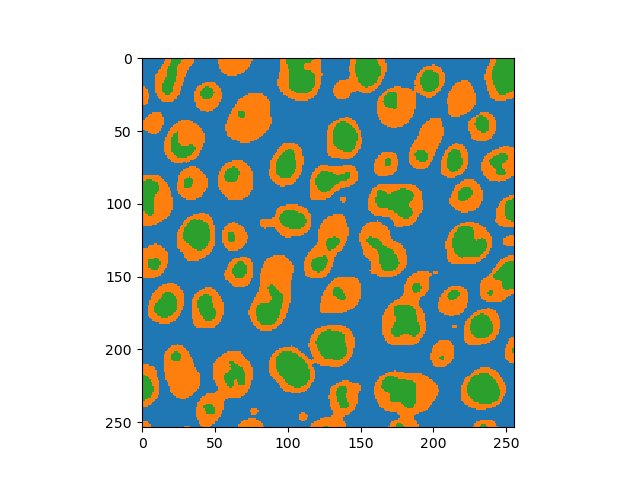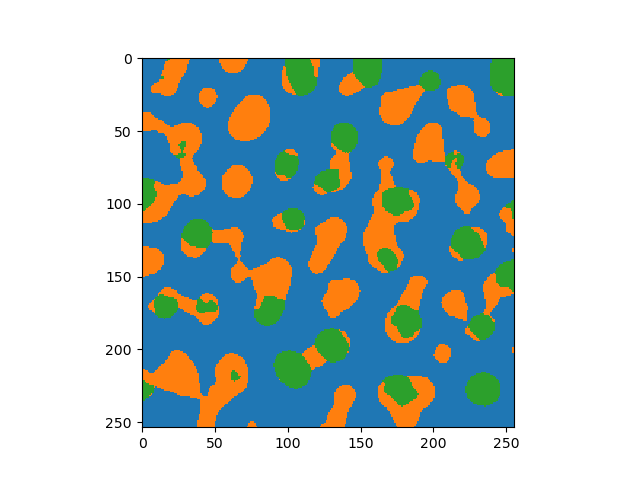# The mode filter for correcting semantic segmentation results#

In descriptive statistics there exist multiple summary measures. The mean and the median filter for example allows locally averaging an image in different ways. The mode filter is less common but still useful in some scenarios. The mode of a pixel in its neighborhood corresponds to the most popular intensity among existing intensities. Thus, it can be used to get rid of indivdual pixels being wrongly classified in a semantic segmentation result.

import numpy as np
import pyclesperanto_prototype as cle
import stackview


For demonstrating the filter, we create a semantic segmentation of blobs.

blobs = cle.imread("../../data/blobs.tif")

semantic_segmentation = (blobs > 70) + \
(blobs > 200) + 1

semantic_segmentation.astype(np.uint32)cle._ image
 shape (254, 256) dtype uint32 size 254.0 kB min 1.0 max 3.0

Using the functions mode_sphere and mode_box we can make the result less noisy.

cle.mode_sphere(semantic_segmentation, radius_x=2, radius_y=2).astype(np.uint32)cle._ image
 shape (254, 256) dtype uint32 size 254.0 kB min 1.0 max 3.0
cle.mode_sphere(semantic_segmentation, radius_x=4, radius_y=4).astype(np.uint32)cle._ image
 shape (254, 256) dtype uint32 size 254.0 kB min 1.0 max 3.0

When the radius becomes wider and wider, the result contains less and less local information.

cle.mode_sphere(semantic_segmentation, radius_x=10, radius_y=10).astype(np.uint32)cle._ image
 shape (254, 256) dtype uint32 size 254.0 kB min 1.0 max 3.0

Tuning the radius manually may help finding a good configuration.

def mode_sphere(image, radius:float = 1):